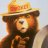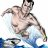# Moving Average Strength Index for ThinkorSwim#### BenTen

Staff
VIP
I want to request a new indicator. It should be histogram, if all conditions meet it should be green and if any one condition does not meet it turns red. You can name indicator as Bingo. Two Requirements:

(1) close > 9 period SMA of the close.
(2) 8 period RSI is more than 14 period RSI and 14 period RSI is more than 19 period RSI.

It should calculate on daily and weekly time frames. Should work on at least stocks, but good to have it work on futures, indices, forex etc. stocks are must requirement. Ideally I want to see when daily turns green from red while weekly is still green in one subgraph if possible..

From what I'm seeing, this indicator (dubbed Bingo by the requester) is similar to the Market Mover Indicator where it utilizes a moving average along with the RSI (Relative Strength Index) to identify a trend.### thinkScript Code

Code:
``````#Bingo

declare lower;

input length1 = 8;

input price = close;
input averageType = AverageType.WILDERS;

def NetChgAvg1 = MovingAverage(averageType, price - price, length1);
def TotChgAvg1 = MovingAverage(averageType, AbsValue(price - price), length1);
def ChgRatio1 = if TotChgAvg1 != 0 then NetChgAvg1 / TotChgAvg1 else 0;

def RSI1 = 50 * (ChgRatio1 + 1);

input length2 = 14;

def NetChgAvg2 = MovingAverage(averageType, price - price, length2);
def TotChgAvg2 = MovingAverage(averageType, AbsValue(price - price), length2);
def ChgRatio2 = if TotChgAvg2 != 0 then NetChgAvg2 / TotChgAvg2 else 0;

def RSI2 = 50 * (ChgRatio2 + 1);
input length3 = 19;

def NetChgAvg3 = MovingAverage(averageType, price - price, length3);
def TotChgAvg3 = MovingAverage(averageType, AbsValue(price - price), length3);
def ChgRatio3 = if TotChgAvg3 != 0 then NetChgAvg3 / TotChgAvg3 else 0;

def RSI3 = 50 * (ChgRatio3 + 1);

input lengthsma = 9;
input displacesma = 0;

def sma = Average(price[-displacesma], lengthsma);

Plot Bingo = RSI1 + SMA/2;
bingo.AssignValueColor(if close > sma && RSI1 > RSI2 && RSI2 > RSI3 then color.green else color.red);
bingo.AssignValueColor(if close > sma && RSI1 > RSI2 && RSI2 > RSI3 then color.green else color.red);
Bingo.SetPaintingStrategy(PaintingStrategy.HISTOGRAM) ;

#Lash``````

https://tos.mx/SDARtf
Credit:

•markosMidpoint Moving Average Indicator Questions 2Moving average cloud? Questions 6ATR Trailing Stop (5atr period.1.5 factor) crossing 50period moving average with Alarm Questions 0